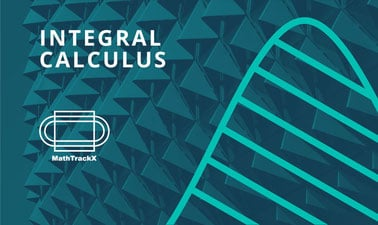# University of Adelaide: MathTrackX: Integral Calculus

Discover concepts and techniques relating to integration and how they can be applied to solve real world problems.4 weeks
3–6 hours per week
Self-paced
Free

This course is part four of the MathTrackX XSeries Program which has been designed to provide you with a solid foundation in mathematical fundamentals and how they can be applied in the real world.

This course will cover basic concepts and techniques relating to integration, another fundamental tool of calculus. Integration is key to understanding the accumulation of a quantity given its rate of change.

Guided by experts from the School of Mathematics and the Maths Learning Centre at the University of Adelaide, this course will cover concepts and techniques to provide a foundation for the applications of differentiation in STEM related careers and/or further study at the undergraduate level.

Join us as we provide opportunities to develop your skills and confidence in applying mathematics to solve real world problems.

### At a glance

• Subject: Math
• Level: Introductory
• Prerequisites:
None
• Language: English
• Video Transcript: English
• Associated programs:
• Associated skills: Calculus, Integration, Integral Calculus, Basic Math

# What you'll learn

Skip What you'll learn
• The concept of anti-derivative as the reverse of differentiation

• How to calculate the anti-derivative of polynomials and special functions

• How to calculate definite integrals and their relation to areas under graphs

• Applications of integration to calculating areas and solving problems in kinematics.